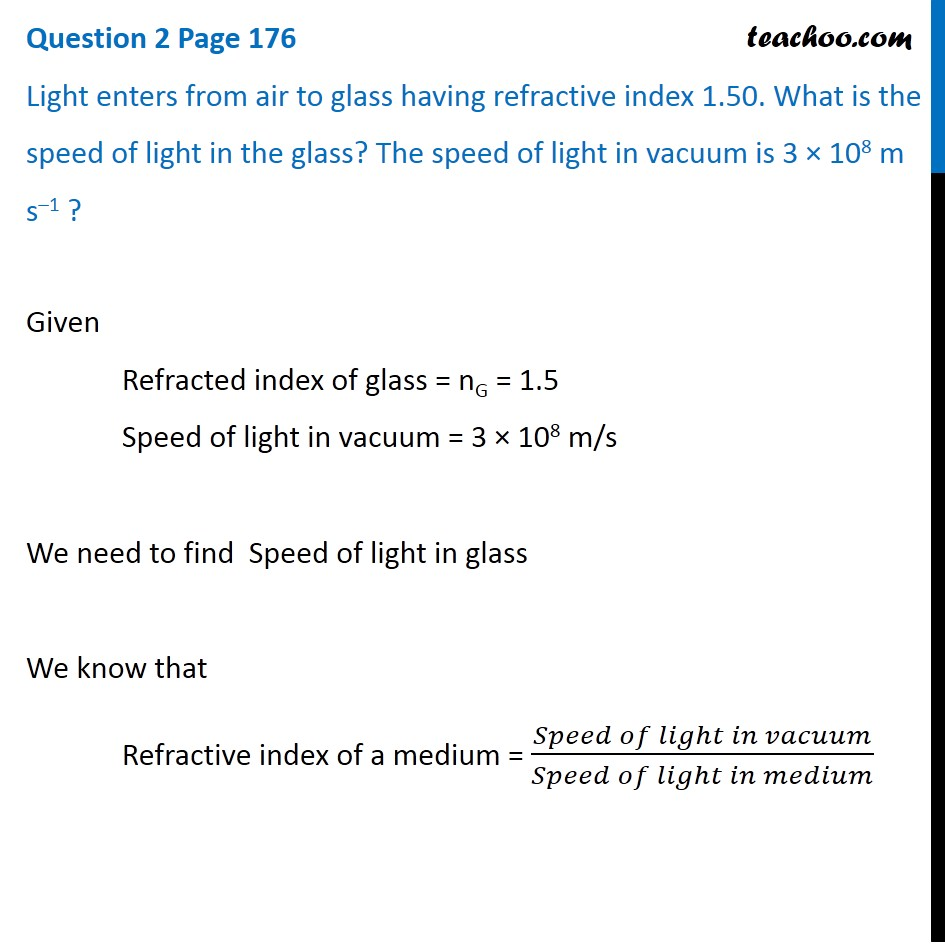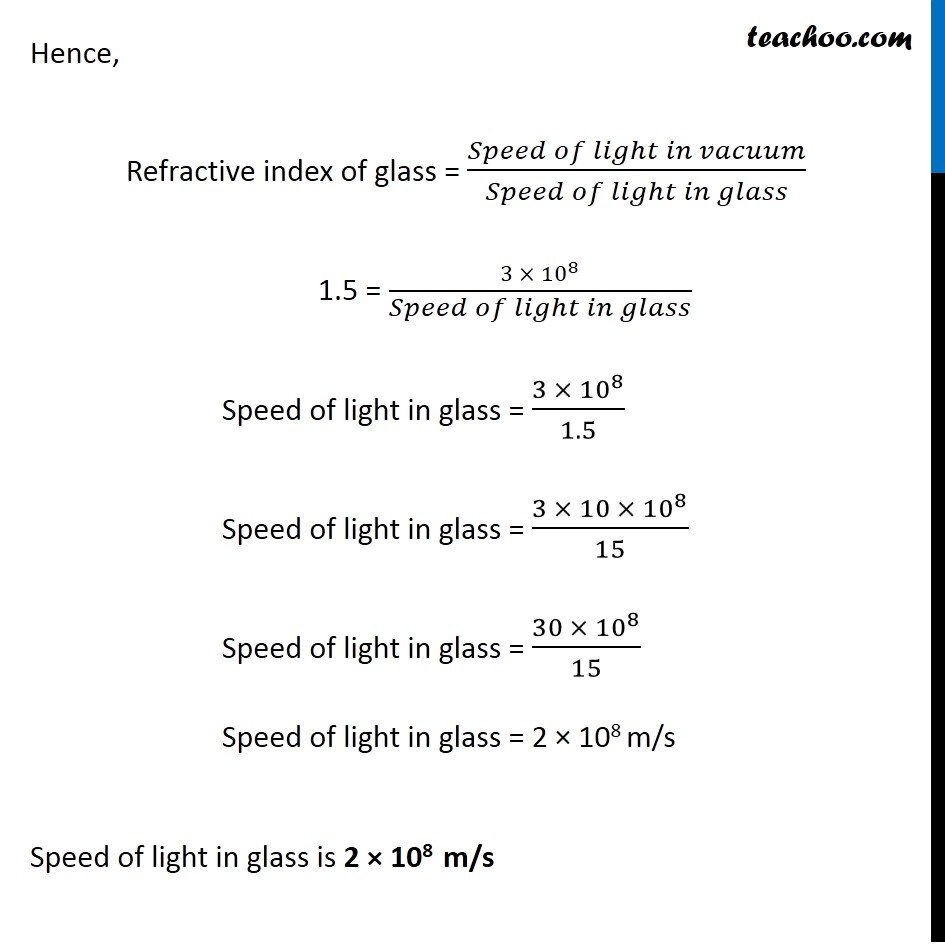Questions from Inside the chapter

Class 10
Chapter 10 Class 10 - Light - Reflection and Refraction

## Light enters from air to glass having refractive index 1.50. What is the speed of light in the glass? The speed of light in vacuum is 3 × 10 8 m s –1Learn in your speed, with individual attention - Teachoo Maths 1-on-1 Class

### Transcript

Question 2 Page 176 Light enters from air to glass having refractive index 1.50. What is the speed of light in the glass? The speed of light in vacuum is 3 × 108 m s–1 ? Given Refracted index of glass = nG = 1.5 Speed of light in vacuum = 3 × 108 m/s We need to find Speed of light in glass We know that Refractive index of a medium = (𝑆𝑝𝑒𝑒𝑑 𝑜𝑓 𝑙𝑖𝑔ℎ𝑡 𝑖𝑛 𝑣𝑎𝑐𝑢𝑢𝑚)/(𝑆𝑝𝑒𝑒𝑑 𝑜𝑓 𝑙𝑖𝑔ℎ𝑡 𝑖𝑛 𝑚𝑒𝑑𝑖𝑢𝑚) Hence, Refractive index of glass = (𝑆𝑝𝑒𝑒𝑑 𝑜𝑓 𝑙𝑖𝑔ℎ𝑡 𝑖𝑛 𝑣𝑎𝑐𝑢𝑢𝑚)/(𝑆𝑝𝑒𝑒𝑑 𝑜𝑓 𝑙𝑖𝑔ℎ𝑡 𝑖𝑛 𝑔𝑙𝑎𝑠𝑠) 1.5 = (3 ×〖 10〗^8)/(𝑆𝑝𝑒𝑒𝑑 𝑜𝑓 𝑙𝑖𝑔ℎ𝑡 𝑖𝑛 𝑔𝑙𝑎𝑠𝑠) Speed of light in glass = (3 × 〖10〗^8)/1.5 Speed of light in glass = (3 × 10 × 〖10〗^8)/15 Speed of light in glass = (30 ×〖 10〗^8)/15 Speed of light in glass = 2 × 108 m/s Speed of light in glass is 2 × 108 m/s